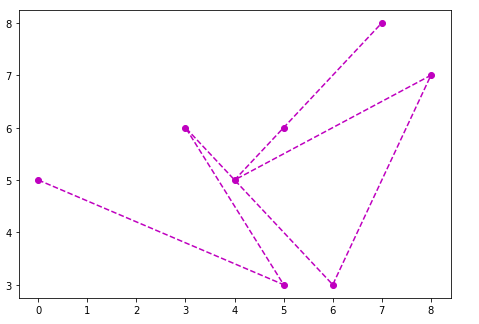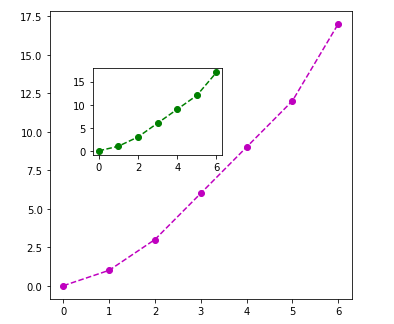# Python | Matplotlib Graph plotting using object oriented API

In object-oriented API, first, we create a canvas on which we have to plot the graph and then we plot the graph. Many people prefer object-oriented API because it is easy to use as compared to functional API.

Let’s try to understand this with some examples.

Example #1:

 `# importing matplotlib library ` `import` `matplotlib.pyplot as plt ` ` `  `# x axis values ` `x ``=``[``0``, ``5``, ``3``, ``6``, ``8``, ``4``, ``5``, ``7``] ` ` `  `# y axis values ` `y ``=``[``5``, ``3``, ``6``, ``3``, ``7``, ``5``, ``6``, ``8``] ` ` `  `# creating the canvas ` `fig ``=` `plt.figure() ` ` `  `# setting the size of canvas ` `axes ``=` `fig.add_axes([``0``, ``0``, ``1``, ``1``]) ` ` `  `# plotting the graph ` `axes.plot(x, y, ``'mo--'``) ` ` `  `# displaying the graph ` `plt.show() `

Output:Everything is pretty much clear in the first example but there is a thing that needs to be focused on, “Setting size of the canvas”, what this basically means is to set the size of the figure on which you want to plot the graph, the syntax is like this.

`add_axes([left, bottom, width, height])`

The values of left, bottom, height and width lies between 0 to 1. Another example will make you more clear about this concept.

Example #2:

 `# importing matplotlib library ` `import` `matplotlib.pyplot as plt ` ` `  `# x-axis values ` `x ``=``[``0``, ``1``, ``2``, ``3``, ``4``, ``5``, ``6``] ` ` `  `# y-axis values ` `y ``=``[``0``, ``1``, ``3``, ``6``, ``9``, ``12``, ``17``] ` ` `  `# creating the canvas ` `fig ``=` `plt.figure() ` ` `  `# setting size of first canvas ` `axes1 ``=` `fig.add_axes([``0``, ``0``, ``0.7``, ``1``]) ` ` `  `# plotting graph of first canvas ` `axes1.plot(x, y, ``'mo--'``) ` ` `  `# setting size of second canvas ` `axes2 ``=` `fig.add_axes([``0.1``, ``0.5``, ``0.3``, ``0.3``]) ` ` `  `# plotting graph of second canvas ` `axes2.plot(x, y, ``'go--'``) ` ` `  `# displaying both graphs ` `plt.show() `

Output:My Personal Notes arrow_drop_upIf you like GeeksforGeeks and would like to contribute, you can also write an article using contribute.geeksforgeeks.org or mail your article to contribute@geeksforgeeks.org. See your article appearing on the GeeksforGeeks main page and help other Geeks.

Please Improve this article if you find anything incorrect by clicking on the "Improve Article" button below.

Article Tags :

Be the First to upvote.

Please write to us at contribute@geeksforgeeks.org to report any issue with the above content.